##### Chemistry For Dummies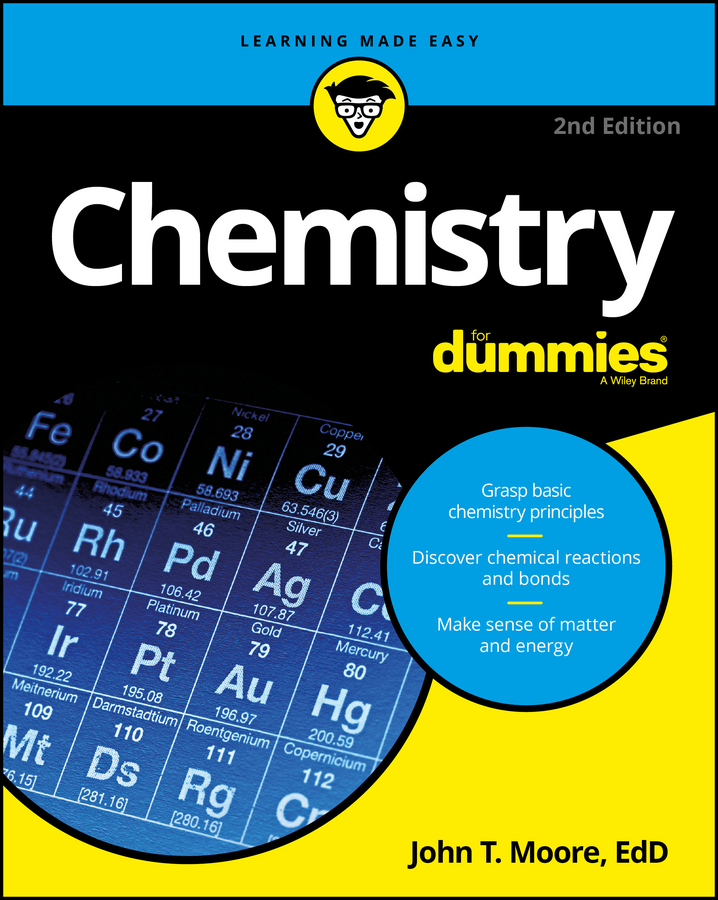You can balance a chemical equation by adjusting the coefficients that precede reactant and product compounds within the equation. After you've got a balanced equation, you can use the coefficients to build mole-mole conversion factors. These kinds of mole conversion factors tell you how much of any given product you get by reacting any given amount of reactant.

Consider the following balanced equation for generating ammonia from nitrogen and hydrogen gases: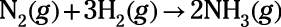Industrial chemists around the globe perform this reaction, humorlessly fixating on how much ammonia product they'll end up with at the end of the day. In any event, how are chemists to judge how closely their reactions have approached completion? The heart of the answer lies in a balanced equation and the mole-mole conversion factors that spring from it.

For every mole of nitrogen reactant, a chemist expects 2 moles of ammonia product. Similarly, for every 3 moles of hydrogen reactant, the chemist expects 2 moles of ammonia product. These expectations are based on the coefficients of the balanced equation and are expressed as mole-mole conversion factors as shown in the figure.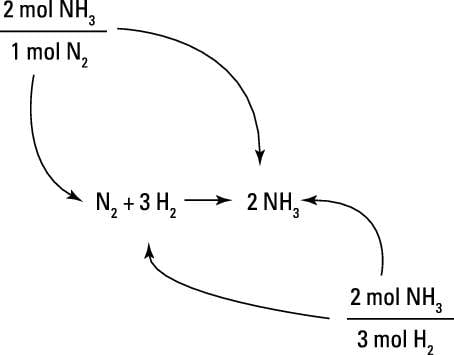Building mole-mole conversion factors from a balanced equation.
For example, say you want to calculate how many moles of ammonia can be expected from the reaction of 278 mol of N2 gas.

To solve this problem, you begin with your known quantity, the 278 mol of nitrogen that's to be reacted. You multiply that quantity by the mole-mole conversion factor that relates moles of nitrogen to moles of ammonia. You write the conversion factor so that mol NH3 is on top and mol N2 is on the bottom. That way, the mol N2 units cancel, leaving you with the desired units, mol NH3. The numbers you put in front of the units for the conversion factor come directly from the coefficients in the balanced chemical equation.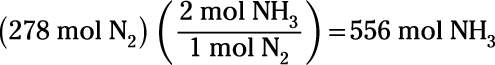So, you expect 556 mol of ammonia from the reaction.

Try another example. One source of hydrogen gas is the electrolysis of water, in which electricity is passed through water to break hydrogen-oxygen bonds, yielding hydrogen and oxygen gases: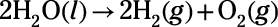Based on this equation, solve these three problems:

1. How many moles of hydrogen gas result from the electrolysis of 78.4 mol of water?

Keep in mind that the number you use for moles is the coefficient for the compound taken from the balanced chemical reaction: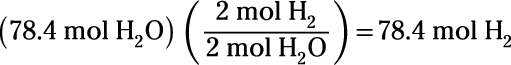You find that the electrolysis reaction produces 78.4 moles of hydrogen gas.

2. How many moles of water are required to produce 905 mol of hydrogen?

To find the moles of water produced, you again apply a mole-mole conversion: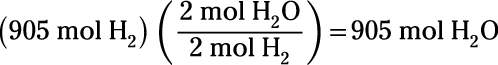This shows you that the reaction produces 905 moles of water.

3. Running the electrolysis reaction in reverse constitutes the combustion of hydrogen. How many moles of oxygen are required to combust 84.6 mol of hydrogen?

Finally, you run the electrolysis reaction in reverse: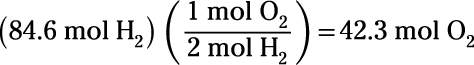This shows that 42.3 moles of oxygen are required to combust 84.6 moles of hydrogen.